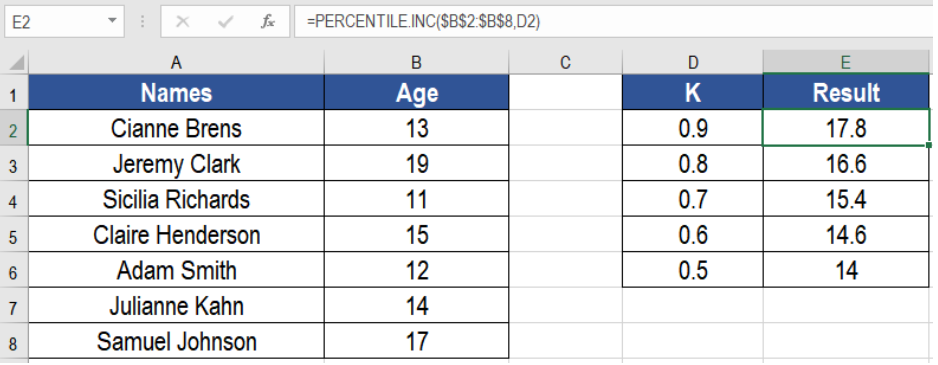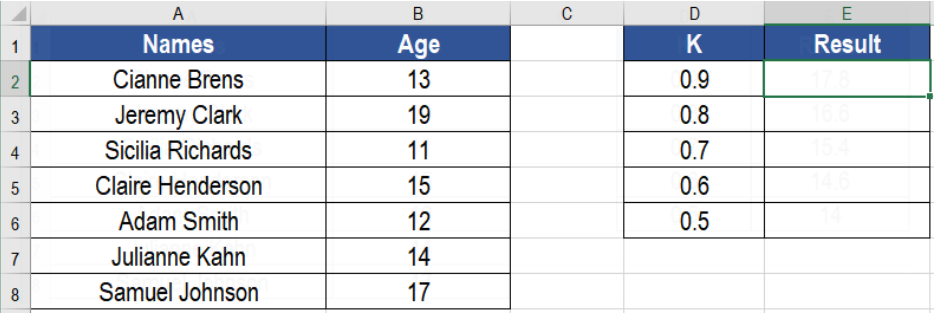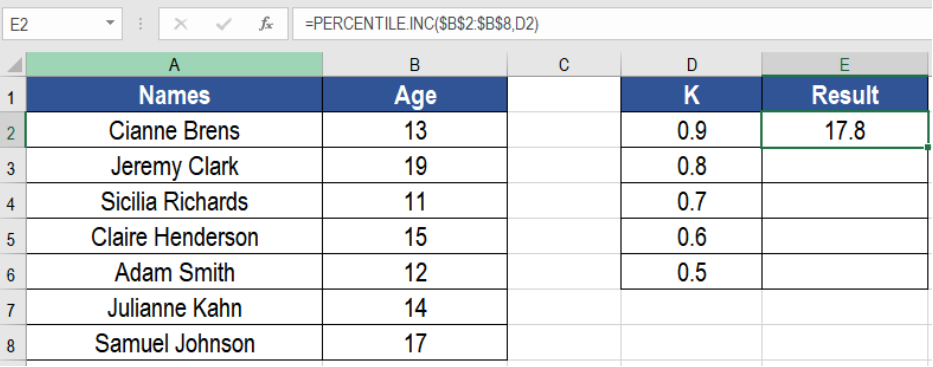Connect to our Experts within seconds for a free diagnosis
Our professional experts are available now. Your privacy is guaranteed.

# Excel PERCENTILE.INC Function

The PERCENTILE.INC function in Excel takes a range and returns the k-th percentile of values. Here, k is a value between 0 and 1, inclusive. It is a great way to calculate percentile from a set of values. In this tutorial, we will learn how to use the PERCENTILE.INC function in Excel.Figure 1. Example of How to Use the Excel PERCENTILE.INC Function

A percentile is a comparison value between a certain value and the values of the rest of the data. For example, say you have scored 80 on a test, and in the 85th percentile. It means that the 80 is higher than 85 % of all other scores. Here, the value for k is 85% or .85.

## Syntax

`=PERCENTILE.INC (array, k)`

## Arguments

• Array – This argument is required. It is the range or set of data values.
• K – This argument is required as well. It is the number in the range 0..1 that represents the kth percentile.

## Setting Up Data

The following example contains a children’s information data set. Column A and B has the names and ages respectively. Column D has the K values.Figure 2. The Data Set

To find out the kth percentile in column E, we need to:

• Go to cell E2.
• Assign the formula `=PERCENTILE.INC(\$B\$2:\$B\$8, D2)` to E2.
• Press Enter.Figure 3. Applying the Formula

• Drag the formula from cells E2 to E5.

This will show the results in column E.

## Notes

1. PERCENTILE.INC returns a #NUM! error if the array is empty.
2. It returns a #VALUE! error if the value for k is non-numeric.
3. PERCENTILE.INC returns a #NUM! error if k is less than 0 or greater than 1.
4. PERCENTILE.INC determines the value at the k-th percentile by interpolation if k is not a multiple of 1/(n – 1).

Most of the time, the problem you will need to solve will be more complex than a simple application of a formula or function. If you want to save hours of research and frustration, try our live Excelchat service! Our Excel Experts are available 24/7 to answer any Excel question you may have. We guarantee a connection within 30 seconds and a customized solution within 20 minutes.